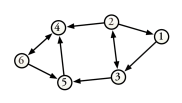# 1.2 Directed Graph

If the edges of $G$ are ordered pairs, then $G$ is a directed graph. In a directed graph, $(v,w)\neq(w,v)$.

A directed graph is often referred to as a digraph. If edge $e$ of a digraph is represented by $(v,w)$, then $e$ is an edge from $v$ to $w$. Vertex $w$ is adjacent to vertex $v$. Vertex $v$ is not adjacent to vertex $w$ unless the edge $(w,v)$ also exists in $G$. The number of vertices adjacent to vertex $v$ is the degree of $v$. In-degree indicates the number of edges incident upon $v$. Out-degree indicates the number of edges emanating from $v$.

Figure 1 depicts a directed graph with six vertices and ten edges. In the figure, the arrowheads indicate the direction of the edge.

Figure 1: Example of a Directed GraphTable 1 summarizes the degree of each vertex in Figure 1.

Table 1: Vertex Degree Summary for Figure 1
 Vertex Degree In-Degree Out-Degree 1 2 1 1 2 4 1 3 3 4 2 2 4 4 3 1 5 3 2 1 6 3 1 2